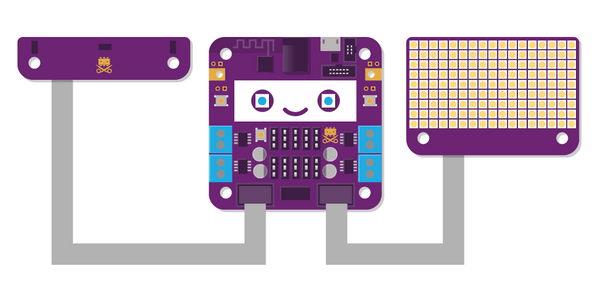# Pong with the Smartibot Distance Sensors and LED Matrix

With this Espruino JavaScript code you can turn the contents of the Smartibot Genius Kit into a tiny console to play the classic 70s computer game. Instead of the dials on the original, this version you move the paddles up and down by moving your hands (or anything else) nearer to, or further away from the two laser distance sensors.This is based heavily on the Pixl.js Pong Game written by Espruino's creator Gordon Williams. The Smartibot version was hacked together as a demo for the Bay Area Maker Faire but it turned out quite fun so we thought other people might want to replicate it. Follow these instructions to get your Smartibot working with the latest version of Espruino and then upload the code below:

``````var smarti = require("Smartibot");

var right = 300;
var left = 300;

var BATSIZE = 2;
var scorel = 0;
var scorer = 0;

var ball;

E.on('init', function() {

var dist = require("Smartibot-distance").connect(smarti.E1);

var g = require("Smartibot-display").connect(smarti.E2);

function newGame() {
ball = {
x : g.getWidth()/2,
y : g.getHeight()/2,
vx : (Math.random()>0.5)?1:-1,
vy : (Math.random()-0.5)*2
};
}

function onFrame() {

right = (right + dist.getRight())/2;

left = (left + dist.getLeft())/2;

var batl = left/250 * g.getHeight();
var batr = right/250 * g.getHeight();

ball.x += ball.vx;
ball.y += ball.vy;
if (ball.y<=0) ball.vy = Math.abs(ball.vy);
if (ball.y>=g.getHeight()-1) ball.vy = -Math.abs(ball.vy);
if (ball.x<5 && ball.y>batl-BATSIZE && ball.y<batl+BATSIZE) {
ball.vx = Math.abs(ball.vx);
}
if (ball.x>g.getWidth()-6  &&
ball.y>batr-BATSIZE && ball.y<batr+BATSIZE) {
ball.vx = -Math.abs(ball.vx);
}
if (ball.x<0) {
scorer++;
newGame();
}
if (ball.x>g.getWidth()-1) {
scorel++;
newGame();
}

g.clear();
g.fillRect(0,batl-BATSIZE, 1,batl+BATSIZE);
g.fillRect(g.getWidth()-2,batr-BATSIZE,             g.getWidth()-1,batr+BATSIZE);
g.fillRect(ball.x,ball.y,ball.x,ball.y);
g.flip();

}

newGame();
setInterval(onFrame, 100);

});
``````